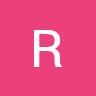Search
•Rob George

# Week 8: 25 Dots Parts D,E,F,G + VCD Interface Sketches

Updated: Dec 10, 2020

## Task 1: 25 Dots Part D

Using for loops to create dots on a screen with small random variations in sizes.float y;

float x;

float diam=50;

float n=120;

void setup() {

size(720, 720);

noLoop();

}

void draw() {

background(255);

noStroke();

fill(0);

//25 Dots

for (x=n; x<width; x=x+n) {

for (y=n; y<height; y=y+n) {

fill(0);

circle(x,y,random(10,60));

}

}

//MATH:

//To Find x and y:

//1) 5/6 = 0.83 2) 720*0.83 = 597.6 (rounded up to 600) 3) 720-600=120

//720/6 = 120

}

## Task 2: 25 Dots Part E

Using for loops to create dots on a screen with larger random variations in sizes.float y;

float x;

float diam=50;

float n=120;

void setup() {

size(720, 720);

noLoop();

}

void draw() {

background(255);

noStroke();

fill(0);

//25 Dots

for (x=n; x<width; x=x+n) {

for (y=n; y<height; y=y+n) {

fill(0);

circle(x,y,random(10,120));

}

}

//MATH:

//To Find x and y:

//1) 5/6 = 0.83 2) 720*0.83 = 597.6 (rounded up to 600) 3) 720-600=120

//720/6 = 120

}

## Task 3: 25 Dots Part F

Using for loops to create dots on a screen. When you roll over the dots they become small dots.

float y;

float x;

float diam=80;

float diam2=10;

float n=120;

void setup() {

size(720, 720);

//noLoop();

}

void draw() {

background(255);

noStroke();

fill(0);

//25 Dots

for (x=n; x<width; x=x+n) {

for (y=n; y<height; y=y+n) {

if (dist(mouseX, mouseY,x,y) > diam){

fill(0);

circle(x,y,diam);

}else{

fill(0);

circle(x,y,diam2);

}

}

}

//MATH:

//To Find x and y:

//1) 5/6 = 0.83 2) 720*0.83 = 597.6 (rounded up to 600) 3) 720-600=120

//720/6 = 120

}

## Task 4: 25 Dots Part G

Using for loops to create dots on a screen. When you roll over the dots they become large dots.

float y;

float x;

float diam=80;

float diam2=10;

float n=120;

void setup() {

size(720, 720);

//noLoop();

}

void draw() {

background(255);

noStroke();

fill(0);

//25 Dots

for (x=n; x<width; x=x+n) {

for (y=n; y<height; y=y+n) {

if (dist(mouseX, mouseY,x,y) < diam){

fill(0);

circle(x,y,diam);

}else{

fill(0);

circle(x,y,diam2);

}

}

}

//MATH:

//To Find x and y:

//1) 5/6 = 0.83 2) 720*0.83 = 597.6 (rounded up to 600) 3) 720-600=120

//720/6 = 120

}

## Assignment 1: Sketches For VCD Promotional Interface

Our next project is a promotional interface design for Montclair State University's Visual Communication Design program. The project is to create an interface that uses 4 words (Experiment, Fail, Learn, Repeat) and use them to visually explain either the program, your experience as a designer in the program, or design in general.

I came up with two concepts for content:

### 1) From My Experience

Experiment: Collaging typography, color, images by clicking and dragging.

Fail: Creating an awful layout for a poster using poorly matched fonts, alignment, and no grid. Each time you click the screen it gets worse.

Learn: Using mouseX and mouseY and mousePressed - it will filter through a set of typography lessons: Kerning, alignment, leading, etc.

Repeat: Images of the Design Process in order repeat on the screen. Hover over to enlarge.

Research=The Red Branding Book and other graphic design books I have

Sketch=my personal sketches for some of my classes

Finalize=A Final Design I created

### 2) Explaining the Program

Experiment: Drawing application and when you click it gives you a task "draw a place" "draw a structure" "draw a location". The next time you open the section it will give you design elements to collage.

Fail: Paper Jam, Paper Cut (Illustrated thumb with paper cut), Paper Torn

Learn: Dots on the screen arranged like the main desk area in the lab. Roll over them and they will enlarge to learn about the faculty, what courses we have, and what to expect.

Repeat: The words research, sketch, mockup and finalize looping in an animation. Every time you click on this section it will be moving in a different direction: either repeating down, up, left, or right with the text color randomly changing but the words always follow the design steps in order.

Tip from critique: Hand rendered type could make it interesting.

### Design Concept 1:

Using the VCD logo as the main page with circular buttons.

Each word has it's own page.

### Design Concept 2:

Using a whiteboard similar to the one in our design studio at Montclair State University. Bookmark as the menu and markers, magnets, and erasers that stick to the board as tools to navigate.

< Back to Coding Main Page